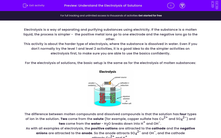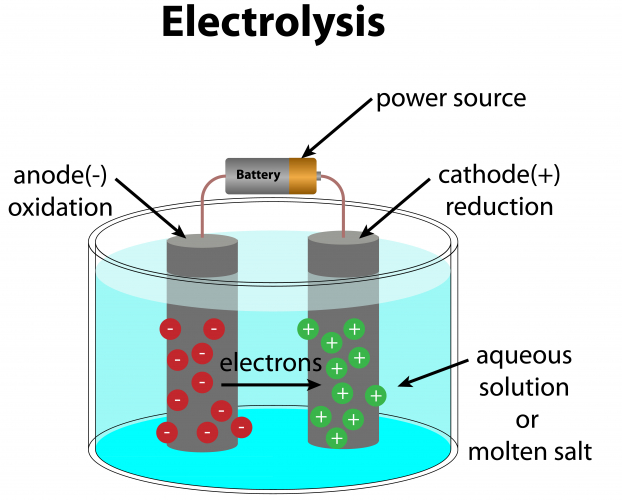# Understand the Electrolysis of Solutions

In this worksheet, students will use ideas of reactivity and electrolysis to predict the products formed from the electrolysis of solutions.Key stage:  KS 4

Year:  GCSE

GCSE Boards:   AQA, AQA Trilogy, AQA Synergy, OCR 21st Century, OCR Gateway, Pearson Edexcel, Eduqas,

Difficulty level:#### Worksheet Overview

Electrolysis is a way of separating and purifying substances using electricity. If the substance is a molten liquid, the process is simpler -  the positive metal ions go to one electrode and the negative ions go to the other.

This activity is about the harder type of electrolysis, where the substance is dissolved in water. Even if you don't normally try the level 1 and level 2 activities, it is a good idea to do the simpler activities on electrolysis first, to make sure you are able to use the basics confidently.

For the electrolysis of solutions, the basic setup is the same as for the electrolysis of molten substances:The difference between molten compounds and dissolved compounds is that the solution has four types of ion in the solution. Two come from the solute (for example, copper sulfate has Cu2+ and SO42-) and two come from the water - H2O breaks down into H+ and OH-

As with all examples of electrolysis, the positive cations are attracted to the cathode and the negative anions are attracted to the anode. So the anode attracts SO42- and OH-, and the cathode attracts Cu2+ and H+

Now comes the super-tricky part. Each electrode is attracting two different ions, so what happens when they reach the electrodes?

What happens at the cathode?

The behaviour of the cathode depends on something similar to the reactivity series. Remember that the best definition of the reactivity series is that more reactive metals push unwanted electrons onto less reactive metals. This means that the electrons at the cathode go on to the ion of the less reactive element. Hydrogen is between copper and iron in the reactivity series, so:

If the metal ion is an unreactive element (copper or below): the metal takes the electrons, and is reduced from metal ions to metal atoms. Hydrogen stays as an ion in the solution.

The half-equation is something like Cu2+ + 2 e- → Cu.

If the metal ion is a reactive element (iron or above): the hydrogen ions take the electrons, and are reduced from hydrogen ions to hydrogen atoms in H2 molecules.  The half-equation is 2H+ + 2 e- → H2.

What happens at the anode?

A similar rule applies to the ions at the anode. The halogens (Group 7) are more reactive than hydroxide, and other anions are less reactive than hydroxide.

If the anion is a halide (for example chloride): the halide gains electrons, and is oxidised from a halide ion to halogen atoms. These atoms become molecules. The half-equation is something like

2Cl- → Cl2 + 2 e-.

If the anion is anything other than a halide (for example sulfate): the hydroxide gains electrons, and is oxidised to form water (which stays in the solution) and oxygen gas.

The half-equation is 4OH- → O2 + 2H2O + 4 e-.

Putting it all together

Suppose we have sodium chloride dissolved in water, NaCl (aq). What happens when we electrolyse this?

1. Work out what the ions are. From the water, we have H+ and OH-. From the sodium chloride, we have Na+ and Cl-.

2. Decide what is attracted to the cathode, and which ion is reduced. H+ and Na+ go to the cathode. Sodium is more reactive than hydrogen, so the hydrogen is reduced. 2H+ + 2 e- → H2 and hydrogen gas is produced.

2. Decide what is attracted to the anion, and which ion is oxidised. OH- and Cl- go to the cathode. Chloride is a halide, so the chloride is oxidised

2Cl- → Cl2 + 2 e- and chlorine gas is produced.

And that's it! This isn't easy - there are lots of steps to think about, and the answers aren't always obvious. But if you think through this step-by-step, you will be able to do it, which will make you officially good at chemistry.

Let's have a go at some questions now.

### What is EdPlace?

We're your National Curriculum aligned online education content provider helping each child succeed in English, maths and science from year 1 to GCSE. With an EdPlace account you’ll be able to track and measure progress, helping each child achieve their best. We build confidence and attainment by personalising each child’s learning at a level that suits them.

Get started